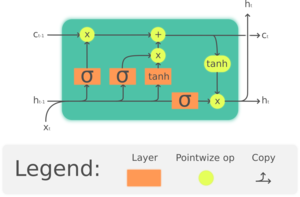# Chapter 12 LSTMCell

So I’m slowly trying to digest what’s going on in the LSTM code in chapter 12. But there are a few things I’m confused about. In the following code, shouldn’t there be a cell state within the LSTMCell object? What’s remembering the state of this LSTMCell if not the cell itself?

``````class LSTMCell(Module):
def __init__(self, ni, nh):
self.forget_gate = nn.Linear(ni + nh, nh)
self.input_gate  = nn.Linear(ni + nh, nh)
self.cell_gate   = nn.Linear(ni + nh, nh)
self.output_gate = nn.Linear(ni + nh, nh)

def forward(self, input, state):
h,c = state
h = torch.stack([h, input], dim=1)
forget = torch.sigmoid(self.forget_gate(h))
c = c * forget
inp = torch.sigmoid(self.input_gate(h))
cell = torch.tanh(self.cell_gate(h))
c = c + inp * cell
out = torch.sigmoid(self.output_gate(h))
h = outgate * torch.tanh(c)
return h, (h,c)
``````

Also I don’t really understand why the return statement is: `return h, (h,c)`. Isn’t that a bit redundant? Why aren’t we just returning `(h,c)`?

Thanks for any clarification!

Hi Gannon,

Thanks for asking an interesting and informative question. If you will let me read between the lines, I am guessing that your confusion is that there are two types of memory in the LSTM.

First, the only state that passes between calls to LSTMCell going forward is the hidden state. Anything that has happened during previous calls to LSTMCell is encoded in it. (The RNN figures out what is pertinent to pass forward, i.e. remember.) This structure is the fundamental nature of an RNN.

At the same time, the internal gates implemented by Linear are learning with each backward pass and weight update. So in that sense, the LSTMCell itself remembers what has been seen during training.

When I was learning about RNNs, I wrote this post to summarize my understanding. It may help you.

As for returning h,(h,c) I don’t know. The standard LSTMCell returns just (h,c). I have not read Chapter 12., so maybe someone familiar with the book will clarify.

To anticipate the next question, PyTorch’s nn.LSTM applies LSTMCell to a whole sequence, giving h and c for each step. It appears to do this all at once and executes much faster than LSTMCell in a loop. But at some level deep inside CUDA it must operate sequentially because RNNs are inherently sequential. So I recommend switching to nn,LSTM whenever possible.

I hope this helps you to get clear and move forward. And if anyone finds a mistake in what I have written, please tell us.

Malcolm1 Like

LSTM Cell is a layer contained in your LSTM model. A cell doesn’t contain state - it does a series operations on hidden state that is contained in the LSTM model (you can see that it is both input and output from the LSTMCell forward method.

On the second question, why do we return h, (h,c) - I think this code is supposed to reflect the LSTM Cell (illustrated in the book) for education purposes - if you check how LSTM is implemented in Pytorch, it will probably be a bit different. That’s just my guess though.

Hi,

In the forward block `h,c=state` refers to the hidden and the cell states being passed as input.

Given that this is a direct implementation of the LSTM cell as the image shown belowIt is understandable that the code returns two hidden states, similar to the depiction.

Your are correct about the redundancy and this is avoided in the base Pytorch code for LSTM.

Note: You can also compare notes for LSTM in d2l book, here they have avoided the redundant hidden state in image representation as well as the code.

I guess what I’m confused about is where the initial state is coming from. Should there be an LSTM class that contains the LSTMCell? In that LSTM class, state would be initialized so we have something to pass to LSTMCell?

I understand that PyTorch’s nn.LSTM is probably way more efficient than anything I could code, but I’m trying to understand the ins and outs of an LSTM by coding it from scratch. But the book seems to skip a step, because I don’t understand how to even pass a state to LSTMCell. In the previous RNNs we save the hidden state within the RNN class, but that’s not the case here.

1 Like

For RNNs are you referring to this part of the code?

``````class LMModel5(Module):
def __init__(self, vocab_sz, n_hidden, n_layers):
self.i_h = nn.Embedding(vocab_sz, n_hidden)
self.rnn = nn.RNN(n_hidden, n_hidden, n_layers, batch_first=True)
self.h_o = nn.Linear(n_hidden, vocab_sz)
self.h = torch.zeros(n_layers, bs, n_hidden)

def forward(self, x):
res,h = self.rnn(self.i_h(x), self.h)
self.h = h.detach()
return self.h_o(res)

def reset(self): self.h.zero_()``````

Yes, LSTMCell processes only a single step of the training sequence. You would define a Module that calls LSTMCell in a loop to process the whole training sequence sequentially. That’s what nn.LSTM does.

The initial hidden state is zeros, AFAICT. It’s fed in to LSTMCell along with the first element of the sequence. I recall (vaguely) that in one of Jeremy’s videos he shows a clever way to write the loop and set the initial state.

…because I don’t understand how to even pass a state to LSTMCell. In the previous RNNs we save the hidden state within the RNN class, but that’s not the case here.

I think you may be confused between LSTMCell and how it is actually used in the loop inside a Module. The hidden state would be retained by the Module and used as input to LSTMCell the next time through the loop.

I can’t speak to the book, but there’s a video (the one mentioned above) where Jeremy develops the code for an RNN in full detail. It would probably explain any missing steps.